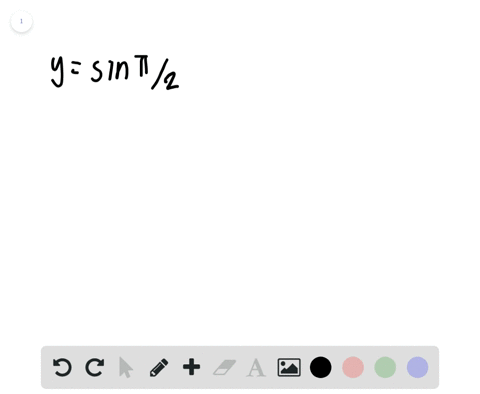🎉 The Study-to-Win Winning Ticket number has been announced! Go to your Tickets dashboard to see if you won! 🎉View Winning Ticket### Sketching the Graph of a Trigonometric Function I…

00:41University of Southern California
Problem 54

# Writing In Exercises 53 and $54,$ use a graphing utility to graph each function $f$ in the same viewing window for $c=-2,$ $c=-1, c=1,$ and $c=2 .$ Give a written description of the change in the graph caused by changing \$c .$$\begin{array}{l}{\text { (a) } f(x)=\sin x+c} \\ {\text { (b) } f(x)=-\sin (2 \pi x-c)} \\ {\text { (c) } f(x)=c \cos x}\end{array}$$

## Discussion

You must be signed in to discuss.

## Video Transcript

this problem asks us to use a graphing utility. I've used Dismas Online Graphing calculator to graph each of the following functions F in the same viewing window for varying values of C negative to negative 11 and two. Then give a written description of the change that you see in the graph caused by changing sea. So for a the function is f of X equals sine X plus C, and you can see that I've created all four graphs and put them on the same plot and what we notices, whereas our base sine function would oscillator around X equals or y equals zero once we add a value outside of our trigger metric function, all of the sine values are shifted vertically by the amount we added. So, for example, in sine X plus one, every single value is shifted up one. And that's why the red one is right here. When you had two, it shifted up to and the same is for the negative eyes is attracting. So as for a written description, I would say varying see causes vertical shift kins, and that's about it frame now moving on to be we see that C is located inside of our trigger metric function, which tells us that our graph is going to be affected by shifts in the ah, horizontal direction. And so, by subtracting the value from the inside, um, we see that our function moves to the right so we can get a visual on this by comparing our blue graph in our red graph Negative sign of two pi X minus one Comparison with negative sign of two pi X minus two. And we see that for that extra value that we subtract that extra one. Our graph shifts to the right, so on the same could be observed when we out of values it shifts to the left. So I would write varying see causes horizontal shifting of our graphs, something like that. And now for sea we see a different change whereas a name B we just had translational changes. Our graphs and see are actually being stretched they're being manipulated. So um and that's changing the amplitude. So we look at our coastline function blue just our standard coast and function And when we multiply buy one has an amplitude of one But when we multiply by two. The amplitude hoops increases. It actually doubles up to two. Um, and this is the same in the negative direction. Um, negative co sign as an amplitude of one and the native to cosign as an amplitude of two. Um, And when we also multiplied by a negative value, we find that our coast and function actually flips to the other side becomes negative. So for this one, I'd write something along the lines of varying see changes, our functions, amplitude and negative. See? Gonna come up here. Values flip r, Graf. And that's it for these variable descriptions of how changing seeing effects are functions output.